# blog posts# Independent t-test or unpaired t-test is one of the statistical parametric tests and its purpose is to find the relationship between two continuous variables in unrelated groups.

For example, comparing height differences between men and women.

## Independent T test assumptions

Before performing the independent t test in SPSS, you should consider the following points:

1. Dependent variables should be measured on a continuous scale (such as distance or relative variables).

2- To perform independent t-test, there must be two dependent variables from two unrelated (independent) groups.

3. There should be no gaps in data collection to evaluate variables.

4- Dependent variables should have a normal distribution.

5. Dependent variables must have the same variance. In other words, the standard deviation of the two dependent variables must be approximately the same. You can use Levin test to evaluate the equality of variance of two variables.

## How to perform independent t-test in SPSS

To better understand how to perform independent t-test in SPSS, we use the example of height difference between men and women.

As you can see in the image below, we have two data columns. The number 1 in the Group column indicates the gender of the man and the number 2 indicates the gender of the woman. The Height column shows the height of each sample. Gender and height are considered as nominal and continuous scales, respectively.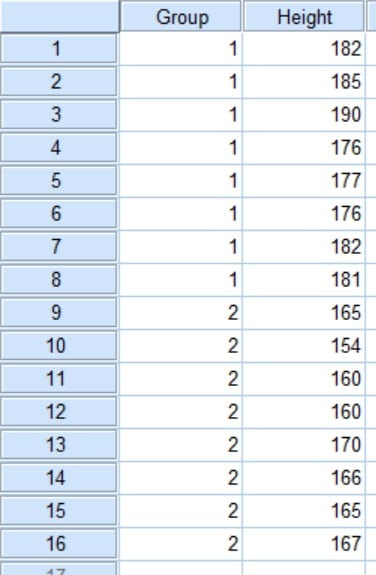The hypotheses of this test are:

“There is no difference between the height of men and women.”

Or

“There is a difference between the height of men and women.”

## Run the test in SPSS

1. First, go to Analyze> Compare Means> Independent-Sample T-Test.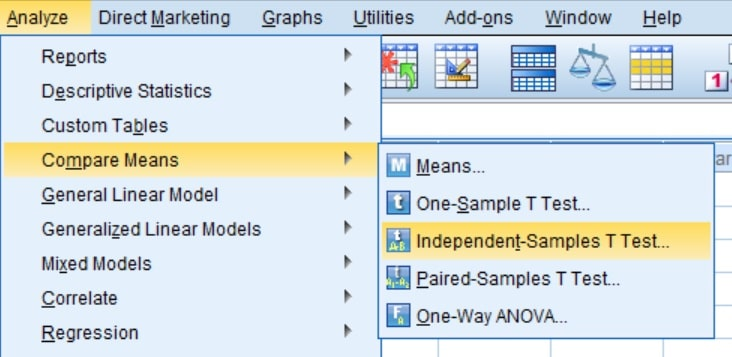2. In the page that opens, in the Test Variable (s) section, you must select the variable name (height) and in the Grouping Variable section, select the group.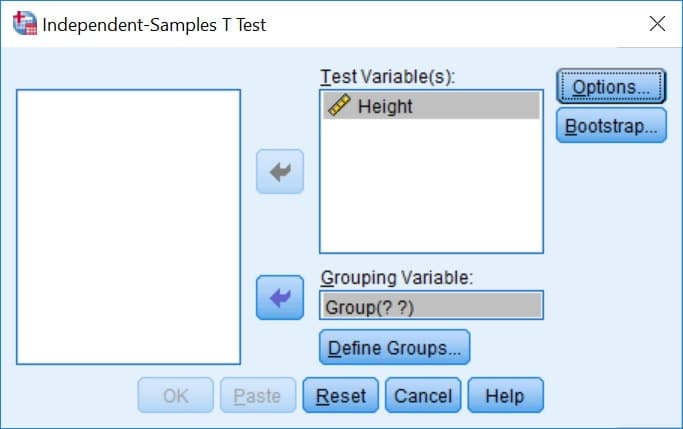3. Then click on the Define Groups option and in the window that opens, enter 1 and 2, respectively, in front of Group 1 and Group 2. In fact, by doing this, you instruct SPSS software to make a comparison between two groups of men (group 1) and women (group 2).4. Click Continue.

5. Then click OK to run the independent T test.

## Output

In the output window, you will see two tables called Group Statistics and Independent Sample Test. The first table provides a series of descriptive information about each of the variables (such as mean, number of samples, and standard deviation). We need the second table to evaluate the test result.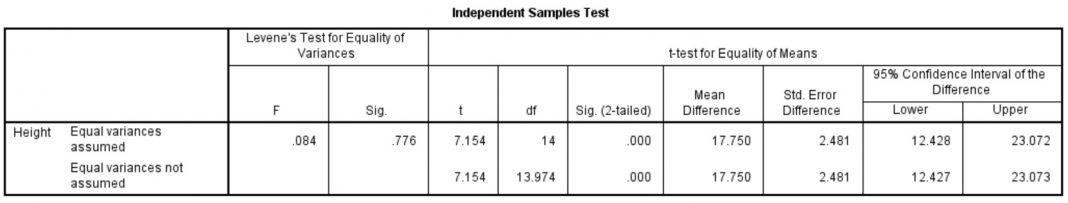With the help of Independent Sample Test table you can get valuable information. for example:

## Levin test for equality of variances

• F – The value of F in the analysis of variance
• Sig.- Significance level of variance equality test

## Independent t-test for equality of means

• t – The value of T
• df – degree of freedom
• Sig. (2-tailed – Significance level of independent t – test in two – sequence analyzes
• Mean Differences – The average difference between two variables
• Error Differences – The standard error for the difference between two variables
• 95% Confidence Interval of the Differences – range of maximum and minimum 95% confidence interval for differences between two variables

## Interpretation of results

Before deciding on a statistical difference, you should be aware of the equality of variance in the data of men and women. To do this, you need to take a look at Levin test results. If the number Sig. If it is more than 0.05, it means that the variance of the two variables is the same, and if it is less than 0.05, it means that the variance of the two variables is not the same.

In this example, the number Sig. Is equal to 0.776, which means that the variance of the two variables is almost the same.

Value 0.000 in the Sig field. (2-tailed) is less than 0.05, which means that there is a significant difference between the height of men and women. Therefore, the first hypothesis is rejected and the second hypothesis is accepted.

## Report results

In writing the report of the independent T test result, it is better to include information about the mean and standard deviation for each variable so that the reader can better understand the difference between the two groups. It is also a good idea to write the value of t, the degree of freedom, and the level of significance at the end of the report. for example:

Men are taller (4.5 181.1 cm) than women (5.1 163.4), 7.154 = (14) t, 0.001 P.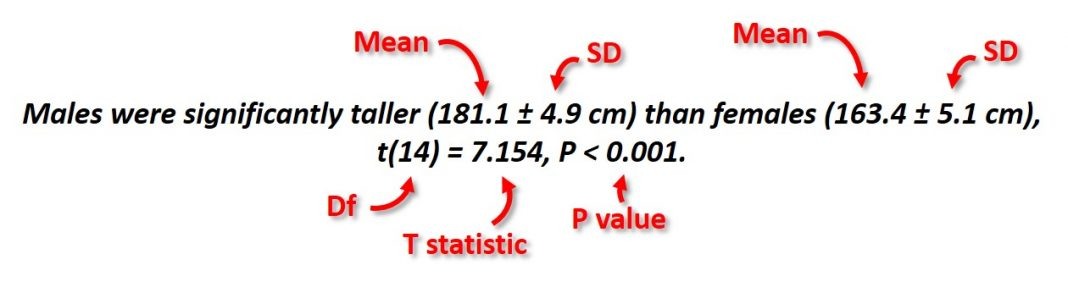• Mean: Mean
• SD: Standard deviation
• Df: degree of freedom
• T Statistics: The value of t
• P Value: Significance level